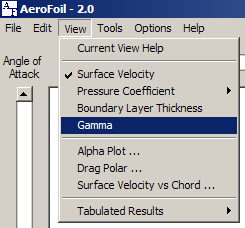## Vortex strength along the airfoil surface (Gamma)AeroFoil used the vortex panel method to calculate ideal flow around the airfoil. The airfoil is approximated by a finite number of line segments. The segments are assumed to be a linear distribution of vortices, whose strengths vary linearly along the length of each panel. The line segments form a closed polygon that is in the shape of an airfoil.

Blue represents a negative vortex (counter-clockwise rotation) and red a positive (clockwise rotation).

The displayed results as a function of the angle of attack are a linear interpolation of the calculated values.

Calculated values along the surface may be examined by scrolling the cursor while holding down the left mouse button.The Display Scale slider may be used to increase or decrease the relative size of the display.